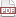### Making integers two-dimensional

22 April 09. [link]PDF version

This is a note about the generally-maligned modulo operation. For the most part, we just use it to get every nth item from a list. Or we might want to shove something into a numerical limit; e.g., I used it the other day for a check digit. The typical check-digit scheme consists of summing a list of elements and then taking that sum mod ten to reduce it to a single digit, or mod 11 to reduce it to a single digit where 10=X.

The use I'll focus on here is in jumping dimensions. Now and then, you find yourself in a linear space, but need to implement two-, three-, or n-dimensional data. Through creative use of the modulo operator, you can easily turn 2-D into 1-D and vice versa.

You'd normally use a double loop to touch every element of a matrix--one loop for the rows and one for the columns. But you can do the same using a single loop and integer division. For int ct = 0, 1, 2, 3, 4, ..., the pair (ct/3, ct%3) takes on the values (0, 0), (0, 1), (0, 2), (1, 0), (1, 1), (1, 2), (2, 0), .... This looks a lot like a double-loop with two variables, and you can use it to cover the same ground. That is, the following two loops behave identically:

    int cols = m->size1;
int rows = m->size2;

for(int i=0; i < rows; i++)
for(int j=0; j < cols; j++)
gsl_matrix_get(m, i, j);

for(int i=0; i < cols * rows; i++)
gsl_matrix_get(m, i/cols, i%cols);


To help you verify this, here's the table that both sets of loops would traverse, with both the one-dimensional index and the coordinate pair:

 i%3 = 0 i%3 = 1 i%3 = 2 i/3 = 0 0 (0, 0) 1 (0, 1) 2 (0, 2) i/3 = 1 3 (1, 0) 4 (1, 1) 5 (1, 2) i/3 = 2 6 (2, 0) 7 (2, 1) 8 (2, 2) i/3 = 3 9 (3, 0) 10 (3, 1) 11 (3, 2)

If you (or your students) are a visual learner, then the mod-as-table form gives you a potentially more comprehensible way to think about an operator with which we have limited day-to-day experience. Once you have the integers in a table, the modulo operation becomes an axis along a space. For example, the condition if ((x % 3) == 1) has a simple physical interpretation: it's just the second column of the table.

Returning to code writing, I'm not presenting this as a clever way to save a line of code: the (int division, modulo) version is typically bad form relative to the simple double-loop. But situations come up reasonably often when you need to put two-dimensional data into a one-dimensional space, and integer arithmetic is the way to do it.

In fact, the pattern continues for more dimensions. Let i be the one-dimensional index the system is handing you, and let dn be the size of the nth dimension in the array you would like to express. Here is the pattern of the coordinates:

 2-D: (i/d1, i%d1) 3-D: (i/(d1*d2), i/d1%d2, i%d1) 4-D: (i/(d1*d2*d3), i/(d1*d2)%d3, i/d1%d2, i%d1) 5-D: (i/(d1*d2*d3*d4), i/(d1*d2*d3)%d4, i/(d1*d2)%d3, i/d1%d2, i%d1)

Next time, a digressive note about integer arithmetic and programming languages.